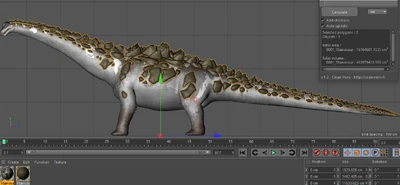25,364 Pages

## Ice Titan Freezes Multiple Titanosaurs.

Feat is here (At 4:55)

Basically the Ice Titan freezes a bunch of Titanosaurs (I counted 5) in one shot. I'll do freezing 1, and 5.

First off we need the mass of a Titanosaur, luckily using the in game models we can find this.
So as the one who changed the size of the titanosaur on this wiki to a more accurate "Roughly 120 metres", I have the exact value it was calculated at, being 119.428535 metres, this will give more accurate stuff so. yeah...
Using cinema 4d I can then find the volume of both the titanosaur and the rocks on it's back seperately (For more accurate results)

## Mass

As you can see in this image (In the little box in the top right), the volume of the body is 7,739,735,938.15cm3

Assuming a similar density to human tissue (there's really not much info I could find on any other creature...) this would make the mass around 7,623,639.89908kgAs we can see in this other image (Same little box), the total volume of the rocks is 453,079,419.565cm3

Assuming the rocks are limestone (which has a density of around 2000kg/m3). would make the mass around 906,158.83913kg

So now we need to know the temperature change, we should be able to find this from what the body temperature of dinosaurs is easy enough

So the change in temperature will be at most 38.2 and at least 35.7 i'll do both for a low and high.
So for the energy to change the temperature of the body we need the specific heat capacity of animal tissue, thanks to omni we can get a value of around 3500J/kg K, and google says that the SHC of Limestone is 0.91kJ/kg K, or 910J/kg K.

Now for the numbers...

E = m*c*ΔT

FOR BODY:
Low end: E = 7623639.89908 * 3500 * 35.7 = 952573805390 Joules
High end: E = 7623639.89908 * 3500 * 38.2 = 1.0192807e+12 Joules

FOR ROCKS:
Low end: 906158.83913 * 910 * 35.7 = 29438382206.8 Joules
High end: 906158.83913 * 910 * 38.2 = 31499893565.8 Joules

TOTAL:
Low end: 29438382206.8 + 952573805390 = 982012187597 = 9.82012187597e+11 Joules = 8-A, Multi-City Block Level
High end: 31499893565.8 + 1.0192807e+12 = 1.0507806e+12 Joules = Still 8-A, Multi-City Block Level

Oh and he froze 5 Titanosaurs not 1 so:
Low end: 9.82012187597e+11 * 5 = 4.9100609e+12J = Low 7-C, Small Town Level
High end: 1.0507806e+12 * 5 = 5.253903e+12J  = Low 7-C, Small Town Level

## Conclusion

### Ice Titan freezing 1 Titanosaur:

Low end: 8-A, Multi-City Block Level

High end: 8-A, Multi-City Block Level

### Ice Titan freezing 5 Titanosaurs:

Low end: Low 7-C, Small Town Level

High end: Low 7-C, Small Town Level

Also the mass of a Titanosaur is 8,529,798.73821kg... Might be useful at some point... I dunno...

Pretty big upgrade altogether either way. Hopefully this can be used.
All other Titans scale to this.

Community content is available under CC-BY-SA unless otherwise noted.